# Betting Best-Of Series

Betting Best of Series is a new expository paper describing the mathematics involved in betting on something like the United States’ Major League Baseball World Series. It isn’t so much about baseball as about demonstrating some of the really great ideas from mathematical finance in a simplified setting. This sort analysis is the “secret sauce” in a lot of financial models and I trying to share the thrilling feeling of working with these techniques in an elementary essay (with diagrams).

Also in (less legible) HTML:

# Betting Best-Of Series

John Mount1

Date: May 27, 2008

# Introduction

We use the United States’ Major League Baseball World Series to demonstrate some of the “arbitrage arguments”2used in mathematical finance. This problem is a classic finance puzzle question and is an interesting introduction to some exciting techniques.

“Arbitrage” is the simultaneous buying and selling of a commodity, usually in multiple markets, that returns a risk-free profit. An example would be finding a market where apples are selling for \$1 and another where they are selling for \$2, and then simultaneously executing a purchase order in the cheap market and a sales order in the expensive market (assuming no significant shipping risks or costs). Typically “arbitrage opportunities” are too much to hope for and to make a profit you must add value, loan money, hold inventory or take on risk. This is just the mathematical finance way of saying “there is no free lunch,” but a number of surprising facts about markets can be proven using this principle.

# The ProblemConsider a “first to win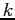” contest like the United States’ Major League Baseball World Series. The World Series is a “first to win four” contest (sometimes called “best of seven”) where a number of games are played between two teams and the first team to win four games is declared the series winner. Ignoring the possibility of ties this process can take from four to seven games. We can (as in Figure 1) lay out all of the possibilities in to a picture that moves from left to right and then moves up when the first team wins and down when the second team wins.

Any sequence of games is represented by a path through this diagram (starting at the left) that reaches a node with no exit. At each node we have marked in the wins for each team (Team One on top, Team Two on the bottom). The nodes where one team has won four games are where the series ends.

The “arbitrage question” is:

If you had access to a bookie who was willing to take an even-payoff bet (on either side) in each game of the World Series, can you design a schedule of bets on games that simulates an even-payoff one dollar bet on the outcome of the entire World Series?

That is: you wish to make a bet that pays you \$1 if your team wins the World Series and costs you \$1 if your team is defeated. You can not find anybody to take such a bet- but you have found a bookie who makes the incredibly generous offer of taking bets (at even pay-off) on each and every game in the series. Can you, without any additional risk, simulate a World Series bet by making a series of per-game bets with this bookie?

The answer turns out to be that you can simulate a world-series bet. The reason for hope is that both types of bets (the even-payoff bets on games and an even-payoff bet on the whole series) are expressing the same underlying belief: that both teams have an exactly equal chance of winning. The teams may or may not have the equal chances of winning- but offering to take bets on both sides at equal pay-off is equivalent expressing just such a belief.

The principle that the probability you are willing to take bets at expresses your subjective probabilities is a principle goes back to Bruno de Finetti and is the most basic “arbitrage style” argument. The principle is simple but it is useful warm-up to think about. Under the assumption that you are “rational” (in the economic sense, which just means you are not giving money away without a reason) and if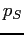denotes your personal estimate of the probability of your team winning then if you are willing to bet \$1 that your team wins at even payoff (meaning you collect \$1 if your team wins pay \$1 if your team loses) then for this bet to make economic sense you must have:which means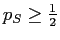.

Similarly if you are willing (for purely economic reasons) to take the other side of the bet at the same even-payoff bet on the other side (reversing the rolls of winning and losing) then it must be true that. We then have our conclusion: from an economic point of view you should be willing to take either side of a fair-payoff bet only if your estimate of the probability of winning is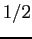.We now return to the World Series diagram. If we bet on individual games (instead of making one bet on the whole series) then at each node in the diagram we expect to have some sort of net winnings or net losses. For example at each node where our team has won four games we should be holding, so we will label these nodes with. Similarly at each node where the opposing team has won for games we expect to have lost exactlyso we label those nodes with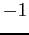. Our task is to figure out the amount bet at each node and our net holdings at each node. If we can find a schedule of bet amounts that leads to the correct outcomes at the end of the world series and starts with an initial net holdings of \$0 then we have solved the problem.

If we look at Figure 2 we see there the node corresponding to each team having won 3 games points to two nodes we know the values of (the World Series ending with either team the winner). We can use the fact that this node points only to nodes with known net holdings to figure out both the bet that must be made at this node and the net holdings this node should have at this point in World Series.

Let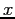be the (unknown) net holdings we have at this node and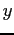be the (unknown) amount we bet then to complete the World Series bet we must have the following: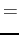This is enough to notice that(your holdings) must be the average of the two outcomes pointed to and(your bet) must be one half of the difference of the two outcomes. So the “each team has won three games” node (near the very right end of the diagram) should have a net holding ofand we should bet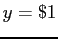. Filling in this node with the net holdings (\$0) now means that there are other nodes that point only to nodes with filled-in net holdings. We can, in fact, repeat this process of filling in each node with unknown net holdings with the average of the two known nodes it points to until we complete the diagram as in Figure 3.In the completed figure each node is filled in with the net holdings required to implement our betting schedule. We can see that a diagram like this can always be filled out to completion by looking at the diagram as having layers like an onion and noticing that we start with the right most nodes filled in (they are the nodes where the world series ends). It is obvious that we can fill out every node in the layer of nodes just inside the outer layer if we start at the right most such node and work back. Every layer can be completed one after another until we get to the inner most layer which is just the starting node. To implement the betting strategy, we keep track of where we are in the diagram and always bet one half of the difference between the net holdings of the two nodes pointed to by the node we are at.

If the first node of the diagram was marked with a value other than zero it would mean that the world-series has a net bias for the first team or the second. Since the rules are symmetric this would be a nonsense conclusion, so we can be sure that all of the even-score nodes must be valued at zero.

The filling in of blanks using values ahead of them (from the future) is the heart of the Binomial Pricing Theory for options is based on a very deep idea called Dynamic Programming. The idea is that you may not know which future you will experience- but you may know the valuation of every possible future. It is an amazing fact that even without introducing probabilities or probability estimates of which future you will experience just knowing the value of every possible future is enough to compute the value of a bet in the present time. In our example: you may not know ahead of time the final scores of the world series, but you do know value of a world series bet for each possible ending score.

# What is the analogy?

From a finance or betting point of view the problem is solved- we have procedures for building the betting schedule and we have the schedule itself. From a mathematician’s point of view we have only just started- we have some procedures and relations but what are they an analogy of?

Naively one might think that they should bet around one fourth of their desired outcome in each game to simulate a best of four series. However to simulate a total World Series bet ofwe use an initial bet of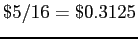in our schedule. This is almost a third of our desired total bet. This gets us wondering: what is the general form of this first bet?

Let
betdenote the amount of the first bet in the simulation of a “best of” bet. If we compute
bet(by constructing betting schedules as above) for many values ofwe see that
betseems to shrink slower than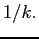In fact it seems to shrink at a rate of around. Even more intriguing if you plotbetbet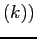it converges (very slowly) to. We can conjecture that for very largethe initial bet is: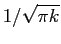whereis the famous ratio of the ratio of the length of the circumference of a circle to the the length of the diameter of the same circle.

Nowis much larger that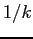(asgets large). So the scheme says to bet a fairly large amount of your budget on the first game, and that winning the first bet is worth a bit more than you would expect (it takes you more than oneth of the way to victory).What is going on? We can again apply an arbitrage or de Finetti style argument and say since the whole game was “fair” with expected pay-off zero then we can relate probabilities and payoffs. The net holdings at each node encode how much of an advantage you have at the node (or how much you should pay to take over from another gambler at this point). If we let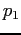denote the probability of going on to win the World Series bet after winning the first bet then we must have: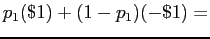bet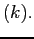Or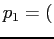bet. For the real World Series we had
bet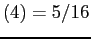so. This means we can read-off from the valuation tree that the probability of winning the World Series (for perfectly equally matched teams) rise fromtoafter you win the first game.3 This can be confirmed from Figure 3. It is easy to confirm that aportion of all paths the node where Team One has one the first game end with Team One winning the whole World Series (each path must be weighted by its probability which are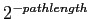). Instead of computing the bets we could have computed the probability of going on to win the World Series at each node4 (and then used the above equivalence principle to read off the required bets).

We can create a new diagram where we start at the node where our team has won the first game and we label all the non-ending nodes with the number of paths that reach the node. For example the two nodes immediately after start can be reach one way each and the next three nodes (“3 games to 0”, “2 games to 1” and “1 games to 2”) can be reached 1,2 and 1 ways respectively. It is a clever trick to notice that the easiest way to count the number of paths to a node is to just add the number of ways found on the previous nodes that point to the our target node. This clever way of counting paths is to use weighted paths (inspired by something called Pascal’s Triangle). Figure 4 shows a few columns of a weighted path diagram (thought he ending nodes are re-written as the sum of the paths reaching them where every path is divided bywhich is the probability of following such a path).

The entries of weighted path diagram are identified by how many columns out from the start node they are and how many steps from one side of the row they are. Both identifiers start at zero so the starting node is denoted as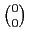the two nodes just after them are denotedand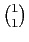. The three nodes just after these are denoted,,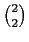and are (as we said before) equal to 1,2 and 1 respectively. These entries are called “binomial coefficients” and the rules for computing them (for integers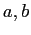) are as follows:

a\$} \
{a choose 0} & = & 1 ;text{if \$a>=0\$} \
{a choose a} & = & 1 ;text{if \$a>=0\$} \
{a choose b} & = & {a-1 choose b-1} + {a-1 choose b} ;text{otherwise.}
end{eqnarray*}
–>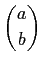if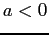or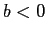or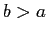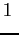ififotherwise.

From our diagram we see that the probability of winning the World Series bet is a diagonal sum across Pascal’s Triangle (weighted by powers of 2). To somebody trained in combinatorics it is obvious5 that a sum like this must itself be a single binomial coefficient. A quick trip to “The On-Line Encyclopedia of Integer Sequences” is enough to identify the solution (Encyclopedia sequence “A001700”) and we can get an exact form for initial bet:

betA lot is known about Binomial coefficients. In fact by a formal called “Stirling’s approximation” we knowas observed.

# Relations

de Finetti used this style of reasoning to provide a foundation for the basic theory of probability. Probability theory has always been somewhat problematic for mathematicians in that it has “content” or “an interpretation” whereas the power of modern mathematics comes from a more axiomatic or content-free way of thinking. The issue is if you are defining the meaning or interpretation of something like probability how do you check or demonstrate that you have the correct meaning without referring to some other pre-existing interpretation? A foundational or first interpretation has trouble looking for prior definitions to show equivalence to.

The arbitrage-free arguments and the binomial arguments in particular are the basis of much of mathematical finance and are the basis for a number of Nobel Prizes in Economics including the Black-Scholes-Merton Option Pricing Model and the Binomial Option Pricing Model.(the ratio of the circumference of a circle to its diameter) is one of the most famous constants in mathematics. Pascal’s Triangle is one of the oldest and most studied diagrams in mathematics with roots all the way back into ancient China. It is actually remarkable how much Zhu Shijie 1303 diagram:looks like our modern version of Pascal’s Triangle (though they are separated by about 350 years, source Wikipedia):. The two diagram differ only in the notation used to write numbers and both start by filling in two diagonals of ones and all other numbers are the sums of the two numbers nearest and above them.

The arguments that replace paths with counts are a particular example of a technique called “Dynamic Programming” invented by Richard Bellman for mathematical optimization and now one of the core concepts of algorithm design.

The idea of using a set of unknown futures that each have a known value is the key idea in solving a number of hard problems in probability and in optimization in the face of uncertainty. One of the the most famous of these problems is the “two armed bandit” where one must decide how to split ones bets between two slot machines that are thought to pay-off at different rates.

For the two armed bandit problem the concern is how long to experiment with both machines when one machine seems to be paying more. The correct solution depends on seeing that how certain you need to be on the difference in machine vales (which in turn drives how long you experiment on both machines). This is a function of how long you intend to use the information. If you intend to play for a long time you want a long initial research phase to produce a very high confidence ranking of the machines; if you do not intend to play for long you want to switch to the machine you suspect is better sooner and on less evidence. Of course “slot machines” is just a toy-problem standing in for uncertain investments, research spending or even spending on different only advertising phrases.

# Conclusions

The finance “no arbitrage” principle is actually a very powerful mathematical tool. It is equivalent to but somewhat more graceful than introducing probabilities when solving some combinatorial problems. In this setting it is equivalent to de Finetti’s principle and converting between probabilities and net holdings is very easy.

## Bibliography

1
BELLMAN, R.
Dynamic Programming.
Dover Publications, 2003.
2
BLACK, F., AND SCHOLES, M.
The pricing of options and corporate liabilities.
The Journal of Political Economy 81, 3 (Jun 1973), 637-654.
3
CHERNOFF, H.
Sequential design of experiments.
Ann. Math. Statist. 30, 3 (Feb 1959), 755-770.
4
COULTER, L. O.
What is mathematics? toward a global view.
17.
5
COX, J. C., ROSS, S. A., AND RUBINSTEIN, M.
Option pricing: A simplified approach.
Journal of Financial Economics (Sep 1979), 39.
6
SHAFER, G., GILLETT, P. R., AND SCHERL, R. B.
A new understanding of subjective probability and its generalization to lower and upper prevision.
Game-Theoretic Probability Project (Oct 2002), 62.

#### Footnotes

… Mount1
http://www.win-vector.com/
… arguments”2
More pedantically we are using the principle of “no arbitrage” or “arbitrage free” argument, but the name is traditional.
… game.3
Again, this if for the unrealistic situation of perfectly matched teams. For teams that have uneven probability the series strongly amplifies the better team’s chance of winning (which is one of the series intents). Also a better could update his subjective probability based on the first outcome which also changes things.
… node4
This calculation is in essence summing end outcomes across all possible paths weighted by how likely each path is. There are many possible paths, but the calculation can be performed quite efficiently.
… obvious5
“Obvious” is actually a special term in mathematics. To illustrate what it means we repeat a story. A mathematician was giving a lecture and stated that the point just shown was obvious. A student asked if it was really obvious. The mathematician stopped the lecture and paused to think. The mathematician thought some more, and eventually walked out of the room. Forty minutes later the mathematician returned to the lecture hall and informed the student that the last point was indeed obvious.### jmount

Data Scientist and trainer at Win Vector LLC. One of the authors of Practical Data Science with R.